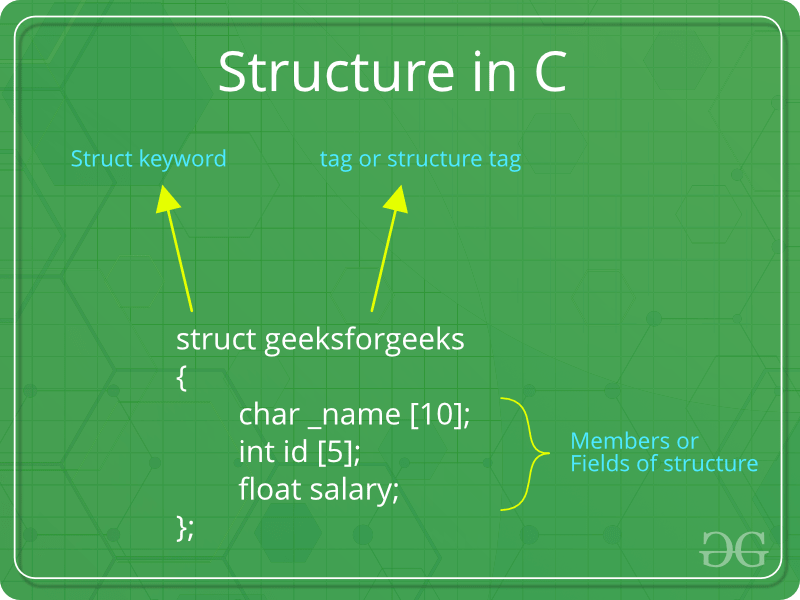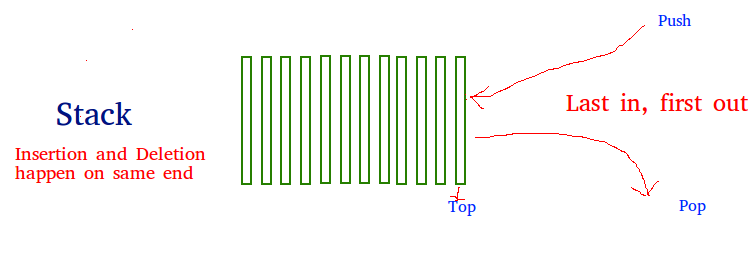# AKTU 1st Year Sem 2 Solved Paper 2016-17 | COMP. SYSTEM & C PROGRAMMING | Sec C

• Last Updated : 28 Jun, 2021

# B.Tech.(SEM-II) THEORY EXAMINATION 2016-17COMPUTER SYSTEM & PROGRAMMING IN C

Time: 3hrs
Total Marks: 100

Note:-

• There are three sections. Section A carries 20 marks, Section B carries 30 marks and Section C carries 50 marks.
• Attempt all questions. Marks are indicated against each question.
• Assume suitable data wherever necessary.

# Section – C

### Attempt any two of the following questions: (2*15 = 30)

3) Define structure with syntax. Also, write a program that compares two given dates. To store date use structure say date that contains three members namely date, month and year. If the dates are equal then display message as “Equal” otherwise “Unequal”.

• A structure is a user-defined data type in C/C++. A structure creates a data type that can be used to group items of possibly different types into a single type.How to create a structure?
‘struct’ keyword is used to create a structure. Following is an example.

 `struct` `address {``    ``char` `name;``    ``char` `street;``    ``char` `city;``    ``char` `state;``    ``int` `pin;``};`

How to declare structure variables?
A structure variable can either be declared with structure declaration or as a separate declaration like basic types.

 `// A variable declaration with structure declaration.``struct` `Point {``    ``int` `x, y;``} p1; ``// The variable p1 is declared with 'Point'`` ` `// A variable declaration like basic data types``struct` `Point {``    ``int` `x, y;``};`` ` `int` `main()``{``    ``struct` `Point p1; ``// The variable p1 is declared like a normal variable``}`

Note: In C++, the struct keyword is optional before in declaration of a variable. In C, it is mandatory.

Program that compares two given dates:

 `#include `` ` `// Declaring the structure of Date``struct` `Date {``    ``int` `date;``    ``int` `month;``    ``int` `year;``};`` ` `// Driver code``int` `main()``{``    ``int` `date1, date2, month1,``        ``month2, year1, year2;`` ` `    ``// Get the first date``    ``scanf``(``"%d"``, &date1);``    ``printf``(``"Enter the first date: %d"``, date1);``    ``scanf``(``"%d"``, &month1);``    ``printf``(``"\nEnter the first month: %d"``, month1);``    ``scanf``(``"%d"``, &year1);``    ``printf``(``"\nEnter the first year: %d"``, year1);`` ` `    ``// Initialise the structure with first date``    ``struct` `Date Date1 = { date1, month1, year1 };`` ` `    ``// Get the second date``    ``scanf``(``"%d"``, &date2);``    ``printf``(``"\nEnter the second date: %d"``, date2);``    ``scanf``(``"%d"``, &month2);``    ``printf``(``"\nEnter the second month: %d"``, month2);``    ``scanf``(``"%d"``, &year2);``    ``printf``(``"\nEnter the second year: %d"``, year2);`` ` `    ``// Initialise the structure with first date``    ``struct` `Date Date2 = { date2, month2, year2 };`` ` `    ``printf``(``"\nThe given dates are: "``);`` ` `    ``// Comparing the Dates``    ``if` `(Date1.date == Date2.date``        ``&& Date1.month == Date2.month``        ``&& Date1.year == Date2.year) {``        ``printf``(``"Equal"``);``    ``}``    ``else` `{``        ``printf``(``"Unequal"``);``    ``}`` ` `    ``return` `0;``}`

Output:

```Enter the first date: 10
Enter the first month: 11
Enter the first year: 2018
Enter the second date: 10
Enter the second month: 11
Enter the second year: 2018
The given dates are: Equal
```
• 4 Write short note on following (Any two):

• (i) Stack with push and pop operation: Stack is a linear data structure which follows a particular order in which the operations are performed. The order may be LIFO(Last In First Out) or FILO(First In Last Out).

Mainly the following three basic operations are performed in the stack:

• Push: Adds an item in the stack. If the stack is full, then it is said to be an Overflow condition.
• Pop: Removes an item from the stack. The items are popped in the reversed order in which they are pushed. If the stack is empty, then it is said to be an Underflow condition.
• Peek or Top: Returns top element of stack.
• isEmpty: Returns true if stack is empty, else false.How to understand a stack practically?
There are many real life examples of stack. Consider the simple example of plates stacked over one another in canteen. The plate which is at the top is the first one to be removed, i.e. the plate which has been placed at the bottommost position remains in the stack for the longest period of time. So, it can be simply seen to follow LIFO/FILO order.

Time Complexities of operations on stack:

push(), pop(), isEmpty() and peek() all take O(1) time. We do not run any loop in any of these operations.

Applications of stack:

Implementation:
There are two ways to implement a stack:

• Using array

Implementing Stack using Arrays

 `// C program for array implementation of stack``#include ``#include ``#include `` ` `// A structure to represent a stack``struct` `Stack {``    ``int` `top;``    ``unsigned capacity;``    ``int``* array;``};`` ` `// function to create a stack of given capacity. It initializes size of``// stack as 0``struct` `Stack* createStack(unsigned capacity)``{``    ``struct` `Stack* stack = (``struct` `Stack*)``malloc``(``sizeof``(``struct` `Stack));``    ``stack->capacity = capacity;``    ``stack->top = -1;``    ``stack->array = (``int``*)``malloc``(stack->capacity * ``sizeof``(``int``));``    ``return` `stack;``}`` ` `// Stack is full when top is equal to the last index``int` `isFull(``struct` `Stack* stack)``{``    ``return` `stack->top == stack->capacity - 1;``}`` ` `// Stack is empty when top is equal to -1``int` `isEmpty(``struct` `Stack* stack)``{``    ``return` `stack->top == -1;``}`` ` `// Function to add an item to stack.  It increases top by 1``void` `push(``struct` `Stack* stack, ``int` `item)``{``    ``if` `(isFull(stack))``        ``return``;``    ``stack->array[++stack->top] = item;``    ``printf``(``"%d pushed to stack\n"``, item);``}`` ` `// Function to remove an item from stack.  It decreases top by 1``int` `pop(``struct` `Stack* stack)``{``    ``if` `(isEmpty(stack))``        ``return` `INT_MIN;``    ``return` `stack->array[stack->top--];``}``// Driver program to test above functions``int` `main()``{``    ``struct` `Stack* stack = createStack(100);`` ` `    ``push(stack, 10);``    ``push(stack, 20);``    ``push(stack, 30);`` ` `    ``printf``(``"%d popped from stack\n"``, pop(stack));`` ` `    ``return` `0;``}`

• (ii) Linked List: Like arrays, Linked List is a linear data structure. Unlike arrays, linked list elements are not stored at contiguous location; the elements are linked using pointers.Arrays can be used to store linear data of similar types, but arrays have following limitations.
1) The size of the arrays is fixed: So we must know the upper limit on the number of elements in advance. Also, generally, the allocated memory is equal to the upper limit irrespective of the usage.
2) Inserting a new element in an array of elements is expensive, because room has to be created for the new elements and to create room existing elements have to shifted.

For example, in a system if we maintain a sorted list of IDs in an array id[].

id[] = [1000, 1010, 1050, 2000, 2040].

And if we want to insert a new ID 1005, then to maintain the sorted order, we have to move all the elements after 1000 (excluding 1000).
Deletion is also expensive with arrays until unless some special techniques are used. For example, to delete 1010 in id[], everything after 1010 has to be moved.

1) Dynamic size
2) Ease of insertion/deletion

Drawbacks:
1) Random access is not allowed. We have to access elements sequentially starting from the first node. So we cannot do binary search with linked lists efficiently with its default implementation. Read about it here.
2) Extra memory space for a pointer is required with each element of the list.
3) Not cache friendly. Since array elements are contiguous locations, there is locality of reference which is not there in case of linked lists.

Representation:
A linked list is represented by a pointer to the first node of the linked list. The first node is called head. If the linked list is empty, then value of head is NULL.
Each node in a list consists of at least two parts:
1) data
2) Pointer (Or Reference) to the next node
In C, we can represent a node using structures. Below is an example of a linked list node with an integer data.

 `// A linked list node``struct` `Node {``    ``int` `data;``    ``struct` `Node* next;``};`

• (iii) Command line argument: The most important function of C/C++ is main() function. It is mostly defined with a return type of int and without parameters :
```int main() { /* ... */ }
```

We can also give command-line arguments in C and C++. Command-line arguments are given after the name of the program in command-line shell of Operating Systems.
To pass command line arguments, we typically define main() with two arguments : first argument is the number of command line arguments and second is list of command-line arguments.

```int main(int argc, char *argv[]) { /* ... */ }
```

or

```int main(int argc, char **argv) { /* ... */ }
```
• argc (ARGument Count) is int and stores number of command-line arguments passed by the user including the name of the program. So if we pass a value to a program, value of argc would be 2 (one for argument and one for program name)
• The value of argc should be non negative.
• argv(ARGument Vector) is array of character pointers listing all the arguments.
• If argc is greater than zero, the array elements from argv to argv[argc-1] will contain pointers to strings.
• Argv is the name of the program, After that till argv[argc-1] every element is command -line arguments.
• 5. What are the different file opening modes in C. Suppose a file contains student’s records with each record containing name and age of a student. Write a C program to read these records and display them in sorted order by name.

• File opening modes in C:
• “r” – Searches file. If the file is opened successfully fopen( ) loads it into memory and sets up a pointer which points to the first character in it. If the file cannot be opened fopen( ) returns NULL.
• “w” – Searches file. If the file exists, its contents are overwritten. If the file doesn’t exist, a new file is created. Returns NULL, if unable to open file.
• “a” – Searches file. If the file is opened successfully fopen( ) loads it into memory and sets up a pointer that points to the last character in it. If the file doesn’t exist, a new file is created. Returns NULL, if unable to open file.
• “r+” – Searches file. If is opened successfully fopen( ) loads it into memory and sets up a pointer which points to the first character in it. Returns NULL, if unable to open the file.
• “w+” – Searches file. If the file exists, its contents are overwritten. If the file doesn’t exist a new file is created. Returns NULL, if unable to open file.
• “a+” – Searches file. If the file is opened successfully fopen( ) loads it into memory and sets up a pointer which points to the last character in it. If the file doesn’t exist, a new file is created. Returns NULL, if unable to open file.

C program to read these records and display them in sorted order by name:

 `// C program to read Student records ``// like id, name and age, ``// and display them in sorted order by Name `` ` `#include  ``#include  ``#include  `` ` `// struct person with 3 fields ``struct` `Student { ``    ``char``* name; ``    ``int` `id; ``    ``char` `age; ``}; `` ` `// setting up rules for comparison ``// to sort the students based on names ``int` `comparator(``const` `void``* p, ``const` `void``* q) ``{ ``    ``return` `strcmp``(((``struct` `Student*)p)->name, ``                ``((``struct` `Student*)q)->name); ``} `` ` `// Driver program ``int` `main() ``{ ``    ``int` `i = 0, n = 5; `` ` `    ``struct` `Student arr[n]; `` ` `    ``// Get the students data ``    ``arr.id = 1; ``    ``arr.name = ``"bd"``; ``    ``arr.age = 12; `` ` `    ``arr.id = 2; ``    ``arr.name = ``"ba"``; ``    ``arr.age = 10; `` ` `    ``arr.id = 3; ``    ``arr.name = ``"bc"``; ``    ``arr.age = 8; `` ` `    ``arr.id = 4; ``    ``arr.name = ``"aaz"``; ``    ``arr.age = 9; `` ` `    ``arr.id = 5; ``    ``arr.name = ``"az"``; ``    ``arr.age = 10; `` ` `    ``// Print the Unsorted Structure ``    ``printf``(``"Unsorted Student Records:\n"``); ``    ``for` `(i = 0; i < n; i++) { ``        ``printf``(``"Id = %d, Name = %s, Age = %d \n"``, ``            ``arr[i].id, arr[i].name, arr[i].age); ``    ``} ``    ``// Sort the structure ``    ``// based on the specified comparator ``    ``qsort``(arr, n, ``sizeof``(``struct` `Student), comparator); `` ` `    ``// Print the Sorted Structure ``    ``printf``(``"\n\nStudent Records sorted by Name:\n"``); ``    ``for` `(i = 0; i < n; i++) { ``        ``printf``(``"Id = %d, Name = %s, Age = %d \n"``, ``            ``arr[i].id, arr[i].name, arr[i].age); ``    ``} `` ` `    ``return` `0; ``} `

Output:

```Unsorted Student Records:
Id = 1, Name = bd, Age = 12
Id = 2, Name = ba, Age = 10
Id = 3, Name = bc, Age = 8
Id = 4, Name = aaz, Age = 9
Id = 5, Name = az, Age = 10

Student Records sorted by Name:
Id = 4, Name = aaz, Age = 9
Id = 5, Name = az, Age = 10
Id = 2, Name = ba, Age = 10
Id = 3, Name = bc, Age = 8
Id = 1, Name = bd, Age = 12
```

• My Personal Notes arrow_drop_up